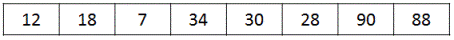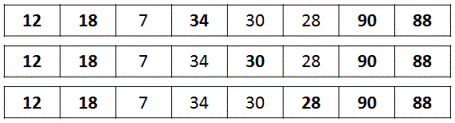# Find the length of longest bitonic subsequence in an array

Given an array of size n, find the longest bitonic subsequence in the array.
A bitonic sequence a sequence which is first increasing and then decreasing.
i.e. it is of the form:
x1, x2, x3, ... xn
where:
x1 < x2 < x3 < ... < xm
xm > xm+1 > xm+2 > ... > xn
For example: 10, 25, 36, 40, 59, 48, 34, 20, 5
Here the sequence first increases from 10 to 59 then descreases to 5.

## Algorithm/Insights

The problem can be solved by using longest increasing subsequence strategy.
Please go through this post on finding longest increasing subsequence:
Longest Increasing Subsequence

Algorithm for finding longest bitonic subsequence:
1. Given input array of length n.
2. Create array lisLength[] where lisLength[i] = Length of Longest Increasing Subsequence in input[0..i] including ith element
3. Create array ldsLength[] where ldsLength[i] = Length of Longest Decreasing Subsequence in input[i..n-1] including ith element
Then length of longest increasing bitonic subsequence which includes the ith element can be calculated as:
lisLength[i] + ldsLength[i] - 1
Here 1 is subtracted from the sum of lengths because ith element is counted twice.
Therefore, length of longest bitonic subsequence is the maximum value for i = 0..n-1
max(lisLength[i] + ldsLength[i] - 1)

Example:
input array:lisLength[] : [1, 0, 0, 0, 0, 0, 0, 0]

i = 1: lisLength = 1
j = 0: input = 12 < input = 18, also lisLength = 1 < lisLength + 1 = 2, so we update:
lisLength = lisLength + 1 = 2
lisLength[] : [1, 2, 0, 0, 0, 0, 0, 0]

i = 2: lisLength = 1
j = 0: input = 12 > input = 7
j = 1: input = 18 > input = 7
lisLength[] : [1, 2, 1, 0, 0, 0, 0, 0]

i = 3: lisLength = 1
j = 0: input = 12 < input = 34, also lisLength = 1 < lisLength + 1 = 2, so we update:
lisLength = lisLength + 1 = 2
j = 1: input = 18 < input = 34, also lisLength = 2 < lisLength + 1 = 3, so we update:
lisLength = lisLength + 1 = 3
j = 2: input =  7 < input = 34, but lisLength = 3 > lisLength + 1 = 2, so lisLength is not updated.
lisLength[] : [1, 2, 1, 3, 0, 0, 0, 0]

i = 4: lisLength = 1
j = 0: input = 12 < input = 30, also lisLength = 1 < lisLength + 1 = 2, so we update:
lisLength = lisLength + 1 = 2
j = 1: input = 18 < input = 30, also lisLength = 2 < lisLength + 1 = 3, so we update:
lisLength = lisLength + 1 = 3
j = 2: input =  7 < input = 30, but lisLength = 3 > lisLength + 1 = 2, so lisLength is not updated.
j = 3: input = 34 > input = 30
lisLength[] : [1, 2, 1, 3, 3, 0, 0, 0]

i = 5: lisLength = 1
j = 0: input = 12 < input = 28, also lisLength = 1 < lisLength + 1 = 2, so we update:
lisLength = lisLength + 1 = 2
j = 1: input = 18 < input = 28, also lisLength = 2 < lisLength + 1 = 3, so we update:
lisLength = lisLength + 1 = 3
j = 2: input =  7 < input = 28, but lisLength = 3 > lisLength + 1, so lisLength is not updated.
j = 3: input = 34 > input = 28
j = 4: input = 30 > input = 28
lisLength[] : [1, 2, 1, 3, 3, 3, 0, 0]

i = 6: lisLength = 1
j = 0: input = 12 < input = 90, also lisLength = 1 < lisLength + 1 = 2, so we update:
lisLength = lisLength + 1 = 2
j = 1: input = 18 < input = 90, also lisLength = 2 < lisLength + 1 = 3, so we update:
lisLength = lisLength + 1 = 3
j = 2: input =  7 < input = 90, but lisLength = 3 > lisLength + 1, so lisLength is not updated.
j = 3: input = 34 < input = 90, also lisLength = 3 < lisLength + 1 = 4, so we update:
lisLength = lisLength + 1 = 4
j = 4: input = 30 < input = 90, but lisLength = 4 = lisLength + 1, so lisLength is not updated.
j = 5: input = 28 < input = 90, but lisLength = 4 = lisLength + 1, so lisLength is not updated.
lisLength[] : [1, 2, 1, 3, 3, 3, 4, 0]

i = 7: lisLength = 1
j = 0: input = 12 < input = 88, also lisLength = 1 < lisLength + 1 = 2, so we update:
lisLength = lisLength + 1 = 2
j = 1: input = 18 < input = 88, also lisLength = 1 < lisLength + 1 = 3, so we update:
lisLength = lisLength + 1 = 3
j = 2: input =  7 < input = 88, but lisLength = 3 > lisLength + 1, so lisLength is not updated.
j = 3: input = 34 < input = 88, also lisLength = 3 < lisLength + 1 = 4, so we update:
lisLength = lisLength + 1 = 4
j = 4: input = 30 < input = 88, but lisLength = 4 = lisLength + 1, so lisLength is not updated.
j = 5: input = 28 < input = 88, but lisLength = 4 = lisLength + 1, so lisLength is not updated.
j = 6: input = 90 > input = 88
lisLength[] : [1, 2, 1, 3, 3, 3, 4, 4]

Similarly, longest decreasing subsequence length array is computed as follows:

ldsLength[] : [0, 0, 0, 0, 0, 0, 0, 1]

i = n-2 = 6: ldsLength = 1
j = n-1 = 7: input = 88 < input = 90
ldsLength = ldsLength + 1 = 2
ldsLength[] : [0, 0, 0, 0, 0, 0, 2, 1]

i = 5: ldsLength = 1
j = 7: input = 88 > input = 28
j = 6: input = 90 > input = 28
ldsLength[] : [0, 0, 0, 0, 0, 1, 2, 1]

i = 4: ldsLength = 1
j = 7: input = 88 > input = 30
j = 6: input = 90 > input = 30
j = 5: input = 28 < input = 30
ldsLength = ldsLength + 1 = 2
ldsLength[] : [0, 0, 0, 0, 2, 1, 2, 1]

i = 3: ldsLength = 1
j = 7: input = 88 > input = 34
j = 6: input = 90 > input = 34
j = 5: input = 28 < input = 34
ldsLength = ldsLength + 1 = 2
j = 4: input = 30 < input = 34
ldsLength = ldsLength + 1 = 3
ldsLength[] : [0, 0, 0, 3, 2, 1, 2, 1]

i = 2: ldsLength = 1
j = 7: input = 88 > input = 7
j = 6: input = 90 > input = 7
j = 5: input = 28 > input = 7
j = 4: input = 30 > input = 7
j = 3: input = 34 > input = 7
ldsLength[] : [0, 0, 1, 3, 2, 1, 2, 1]

i = 1: ldsLength = 1
j = 7: input = 88 > input = 18
j = 6: input = 90 > input = 18
j = 5: input = 28 > input = 18
j = 4: input = 30 > input = 18
j = 3: input = 34 > input = 18
j = 2: input =  7 < input = 18
ldsLength = ldsLength + 1 = 2
ldsLength[] : [0, 2, 1, 3, 2, 1, 2, 1]

i = 0: ldsLength = 1
j = 7: input = 88 > input = 12
j = 6: input = 90 > input = 12
j = 5: input = 28 > input = 12
j = 4: input = 30 > input = 12
j = 3: input = 34 > input = 12
j = 2: input =  7 < input = 12
ldsLength = ldsLength + 1 = 2
j = 1: input = 18 > input = 12
ldsLength[] : [2, 2, 1, 3, 2, 1, 2, 1]

lisLength[] : [1, 2, 1, 3, 3, 3, 4, 4]
ldsLength[] : [2, 2, 1, 3, 2, 1, 2, 1]

Finally, length of longest bitonic subsequence is calculated:
lisLength + ldsLength - 1  =  2 + 2 - 1  =  3
lisLength + ldsLength - 1  =  1 + 1 - 1  =  1
lisLength + ldsLength - 1  =  3 + 3 - 1  =  5
lisLength + ldsLength - 1  =  3 + 2 - 1  =  4
lisLength + ldsLength - 1  =  3 + 1 - 1  =  3
lisLength + ldsLength - 1  =  4 + 2 - 1  =  5    ---> MAXIMUM
lisLength + ldsLength - 1  =  4 + 1 - 1  =  4

Hence, solution = 5
As can be seen from any of the following bitonic subsequences.## Code Snippet

```
package com.ideserve.questions.saurabh;

/**
* <b>IDeserve <br>
* Find the length of longest bitonic subsequence in an array
*
* @author Saurabh
*/
public class LongestBitonicSubsequence {

public static int longestBitonicSubsequence(int[] input) {

if(input == null || input.length == 0) {
return 0;
}

int n = input.length;
// lisLength[i] = Length of Longest Increasing Subsequence in input[0..i]
int[] lisLength = new int[n];
// ldsLength[i] = Length of Longest Increasing Subsequence in input[i..n-1]
int[] ldsLength = new int[n];
int maxLength = 1;

// Find lengths of longest increasing subsequence for all sub arrays [0..i]
lisLength = 1;
for (int i = 1; i < n; i++) {
lisLength[i] = 1;
for (int j = 0; j < i; j++) {
if (input[i] > input[j] && lisLength[i] < lisLength[j] + 1) {
lisLength[i] = lisLength[j] + 1;
}
}
}

// Find lengths of longest decreasing subsequence for all sub arrays [i..n-1]
ldsLength[n-1] = 1;
for (int i = n - 2; i >= 0; i--) {
ldsLength[i] = 1;
for (int j = n - 1; j > i; j--) {
if (input[i] > input[j] && ldsLength[i] < ldsLength[j] + 1) {
ldsLength[i] = ldsLength[j] + 1;
}
}
}

for (int i = 1; i < n; i++) {
if (maxLength < lisLength[i] + ldsLength[i] - 1)
maxLength = lisLength[i] + ldsLength[i] - 1;
}

return maxLength;
}

public static void main(String[] args) {
int[] array = { 12, 18, 7, 34, 30, 28, 90, 88 };
System.out.println("Length of Longest Bitonic Subsequence: " + longestBitonicSubsequence(array));
}
}
```

## Order of the Algorithm

Time Complexity is O(n^2)
Space Complexity is O(n)